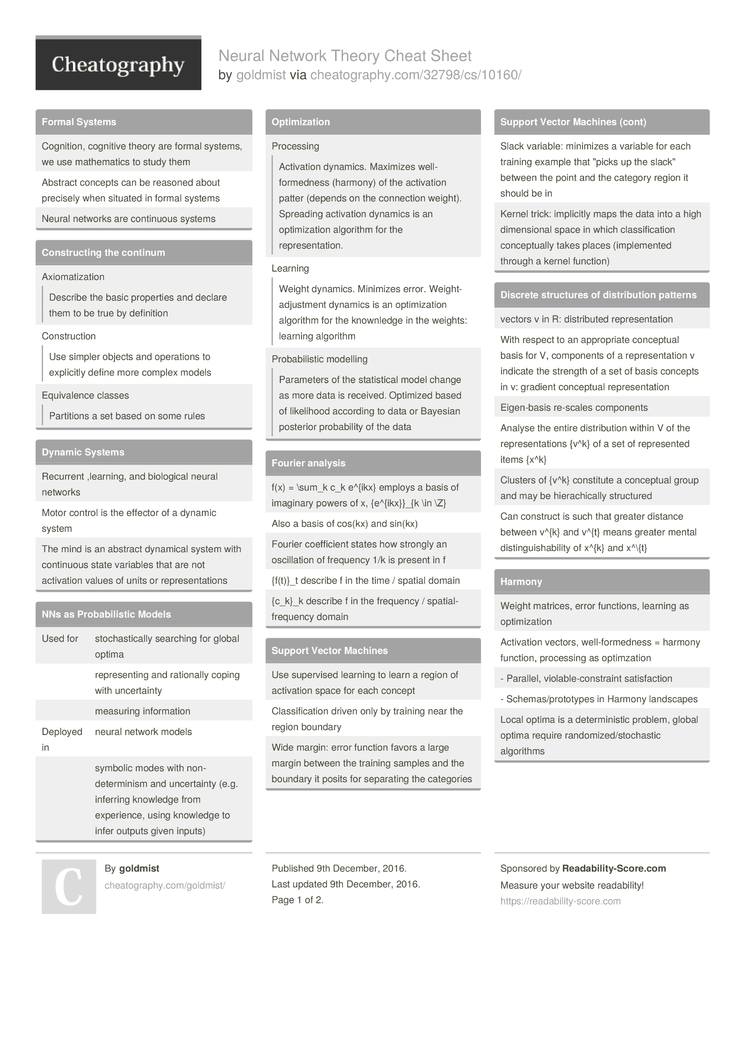# Neural Network Theory Cheat Sheet by goldmist

### Formal Systems

 Cognition, cognitive theory are formal systems, we use mathem­atics to study them Abstract concepts can be reasoned about precisely when situated in formal systems Neural networks are continuous systems

### Constr­ucting the continum

 Axioma­tiz­ationDescribe the basic properties and declare them to be true by definition Constr­uctionUse simpler objects and operations to explicitly define more complex models Equiva­lence classesPartitions a set based on some rules

### Dynamic Systems

 Recurrent ,learning, and biological neural networks Motor control is the effector of a dynamic system The mind is an abstract dynamical system with continuous state variables that are not activation values of units or repres­ent­ations

### NNs as Probab­ilistic Models

 Used for stocha­sti­cally searching for global optima repres­enting and rationally coping with uncert­ainty measuring inform­ation Deployed in neural network models symbolic modes with non-de­ter­minism and uncert­ainty (e.g. inferring knowledge from experi­ence, using knowledge to infer outputs given inputs)

### Optimi­zation

 ProcessingActivation dynamics. Maximizes well-f­orm­edness (harmony) of the activation patter (depends on the connection weight). Spreading activation dynamics is an optimi­zation algorithm for the repres­ent­ation. LearningWeight dynamics. Minimizes error. Weight­-ad­jus­tment dynamics is an optimi­zation algorithm for the knownledge in the weights: learning algorithm Probab­ilistic modellingParameters of the statis­tical model change as more data is received. Optimized based of likelihood according to data or Bayesian posterior probab­ility of the data

### Fourier analysis

 f(x) = \sum_k c_k e^{ikx} employs a basis of imaginary powers of x, {e^{i­kx}}_{k \in \Z} Also a basis of cos(kx) and sin(kx) Fourier coeffi­cient states how strongly an oscill­ation of frequency 1/k is present in f {f(t)}_t describe f in the time / spatial domain {c_k}_k describe f in the frequency / spatia­l-f­req­uency domain

### Support Vector Machines

 Use supervised learning to learn a region of activation space for each concept Classi­fic­ation driven only by training near the region boundary Wide margin: error function favors a large margin between the training samples and the boundary it posits for separating the categories Slack variable: minimizes a variable for each training example that "­picks up the slack" between the point and the category region it should be in Kernel trick: implicitly maps the data into a high dimens­ional space in which classi­fic­ation concep­tually takes places (imple­mented through a kernel function)

### Discrete structures of distri­bution patterns

 vectors v in R: distri­buted repres­ent­ation With respect to an approp­riate conceptual basis for V, components of a repres­ent­ation v indicate the strength of a set of basis concepts in v: gradient conceptual repres­ent­ation Eigen-­basis re-scales components Analyse the entire distri­bution within V of the repres­ent­ations {v^k} of a set of repres­ented items {x^k} Clusters of {v^k} constitute a conceptual group and may be hierac­hically structured Can construct is such that greater distance between v^{k} and v^{t} means greater mental distin­gui­sha­bility of x^{k} and x^\{t}

### Harmony

 Weight matrices, error functions, learning as optimi­zation Activation vectors, well-f­orm­edness = harmony function, processing as optimz­ation - Parallel, violab­le-­con­straint satisf­action - Schema­s/p­rot­otypes in Harmony landscapes Local optima is a determ­inistic problem, global optima require random­ize­d/s­toc­hastic algorithms

### Inductive learning

 Finding the best hypothesis within a hypothesis space about the word that the learning is trying to understand Goodness of a hypothesis is determined jointly by how well H fits the data that the learning has received about the world and how simple H is A hypothesis is a probab­ilistic data-g­ene­rator Maximum Likelihood Principle Maximum A Posteriori Principle: Bayesian principle that says pick the hypothesis that has highest a posteriori probab­ility, balancing the likelihood of the data against the a priori probab­ility of H. Best not pick a H, maintain a degree of belief for every H in the H space Maximum Entropy Principle: Maxent. Pick the H with the max missing inform­ation, among those H that are consistent with the known data Minimum Descri­ption Length Principle: shorter is better

## Download the Neural Network Theory Cheat Sheet2 Pages
//media.cheatography.com/storage/thumb/goldmist_neural-network-theory.750.jpg

PDF (recommended)